# Lattice of points

point lattice, in, with basisThe set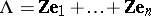of points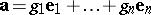, whereare integers.

The latticecan be regarded as the free Abelian group withgenerators. A lattice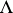has an infinite number of bases; their general form is, whereruns through all integral matrices of determinant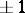. The quantity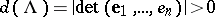is the volume of the parallelopipedon formed by the vectors. It does not depend on the choice of a basis and is called the determinant of the lattice.

The partition of point lattices into Voronoi lattice types plays an important role in the geometry of quadratic forms (cf. Quadratic form).